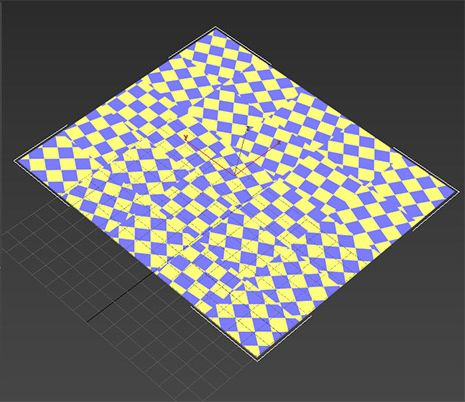# Base::rotation_translation_scale and base::transform_coordinate require uniform parameters?

I am trying to use base::transform_coordinate to rotate uvw coordinates.
This works fine if i use uniform values for the entire geometry, but i would like to use different rotations for different sections (think grid tiles) of the uvw range. This causes an error with the compiler. The documentation indicates that these can be varying, if i read it correctly. I would be very grateful if someone could point out what i am missing here (and/or an alternative way of achieving an effect like this in MDL)(5x5 tiles of a pattern/texture with randomized rotation)

This is my code

``````export float rand(float seed)
{
return math::frac(math::sin(seed) * 43758.5453123);
}

export float randomFloatInRange(float minValue, float maxValue, float seed)
{
float range = maxValue - minValue;
return rand(seed) * range + minValue;
}

base::texture_coordinate_info rotate_grid_tiles(base::texture_coordinate_info uvw, uniform float seed)
{
//convert to integers to make 1-unit-sized grid tiles
int2 gridIndex = int2(int(uvw.position.x), int(uvw.position.y));

//calculate randomized rotation matrix for each grid cell
//NOTE: Fails to compile, works with fixed rotation parameter
float4x4 tile_rotation = base::rotation_translation_scale(rotation: float3(0,0, randomFloatInRange(0, 90, seed + gridIndex + gridIndex)));

base::texture_coordinate_info uvw_rotated = base::transform_coordinate(
transform: tile_rotation,
coordinate: uvw_tiles);

return uvw_rotated;
}
``````

The errors generated are:

``````> mosaic_test.mdl(29,63): C220 'rotation_translation_scale' : cannot convert first argument from 'float3' to 'uniform float3': depends on parameter type
> mosaic_test.mdl(32,9): C220 'transform_coordinate' : cannot convert first argument from 'float4x4' to 'uniform float4x4': depends on parameter type
``````

This has recently been changed, so this depends on the version of the SDK you are using.
MDL SDK 2022.1, build 363600.1420 has varying transforms in ::base

if you need to work with an older OV/Iray/MDL SDK, you can copy the new base.mdl and rename it and use that one. (or just copy the relevant functions to your own module)

has the restriction removed

1 Like

This topic was automatically closed 14 days after the last reply. New replies are no longer allowed.